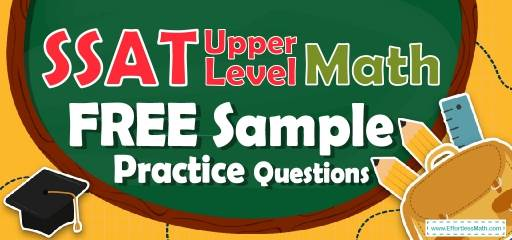# SSAT Upper Level Math FREE Sample Practice QuestionsPreparing for the SSAT Upper-Level Math test? To do your best on the SSAT Upper-Level Math test, you need to review and practice real SSAT Upper-Level Math questions.  There’s nothing like working on SSAT Upper-Level Math sample questions to hone your math skills and put you more at ease when taking the SSAT Upper-Level Math test. The sample math questions you’ll find here are brief samples designed to give you the insights you need to be as prepared as possible for your SSAT Upper-Level Math test.

Check out our sample SSAT Upper-Level Math practice questions to find out what areas you need to practice more before taking the SSAT Upper-Level Math test!

Start preparing for the 2022 SSAT Upper-Level Math test with our free sample practice questions. Also, make sure to follow some of the related links at the bottom of this post to get a better idea of what kind of mathematics questions you need to practice.

## 10 Sample SSAT Upper-Level Math Practice Questions

1- The sum of six different negative integers is $$-80$$. If the smallest of these integers is $$-16$$, what is the largest possible value of one of the other five integers?

A. $$-16$$

B. $$-10$$

C. $$-8$$

D. $$-4$$

E. $$-1$$

2- What is the slope of a line that is perpendicular to the line $$2x-4y=24$$?

A. $$-2$$

B. $$-\frac{1}{2}$$

C. 6

D. 12

E. 14

3- The width of a box is one-third of its length. The height of the box is one-half of its width. If the length of the box is $$24$$ cm, what is the volume of the box?

A. $$91$$ cm$$^3$$

B. $$172$$ cm$$^3$$

C. $$254$$ cm$$^3$$

D. $$768$$ cm$$^3$$

E. $$2,990$$ cm$$^3$$

4- A football team won exactly $$70\%$$ of the games it played during the last session. Which of the following could be the total number of games the team played last season?

A. 59

B. 45

C. 72

D. 20

E. 11

5- The Jackson Library is ordering some bookshelves. If $$x$$ is the number of bookshelves the library wants to order, which each cost $$\200$$ and there is a one-time delivery charge of $$\600$$, which of the following represents the total cost, in the dollar, per bookshelf?

A. $$\frac{200x+600}{x}$$

B. $$\frac{200x+600}{600}$$

C. $$200+600x$$

D. $$200x+600$$

E. $$200x-600$$

6- There are $$14$$ marbles in bag A and $$18$$ marbles in bag B. If the sum of the marbles in both bags will be shared equally between two children, how many marbles bag A has less than the marbles that each child will receive?

A. 2

B. 3

C. 4

D. 5

E. 6

7- If Jason’s mark is F more than Alex’s, and Jason’s mark is $$18$$, which of the following can be Alex’s mark?

A. $$18-F$$

B. $$F-18$$

C. $$\frac{F}{18}$$

D. $$18F$$

E. $$18+F$$

8- When number $$81,602$$ is divided by $$240$$, the result is closest to?

A. 4

B. 30

C. 200

D. 300

E. 340

9- The price of a sofa is decreased by $$50\%$$ to $$\530$$. What was its original price?

A. $580 B.$620

C. $760 D.$900

E. $1,060 10- If the perimeter of the following figure be $$33$$, what is the value of $$x$$? $$\img{https://appmanager.effortlessmath.com/public/images/questions/dfgfgh.png }$$ A. 3 B. 4 C. 6 D. 8 E. 11 ## Best SSAT Upper-Level Math Prep Resource for 2022 ## Answers: 1- B The smallest number is $$-16$$. To find the largest possible value of one of the other five integers, we need to choose the smallest possible integers for four of them. Let $$x$$ be the largest number. Then: $$-80=(-16)+(-15)+(-14)+(-13)+(-12)+x→$$ $$-80=-70+x , →x=-80+70=-10$$ 2- A The equation of a line in slope intercept form is: $$y=mx+b$$, Solve for $$y$$. $$2x-4y=24⇒-4y=24-2x⇒y=(24-2x)÷(-4)⇒y=\frac{1}{2} x-6$$ The slope is $$\frac{1}{2}$$. The slope of the line perpendicular to this line is: $$m_{1}×m_{2}= -1⇒\frac{1}{2} ×m_{2}=-1⇒m_{2}=-2$$ 3- D If the length of the box is $$24$$, then the width of the box is one-third of it, $$8$$, and the height of the box is $$4$$ (half of the width). The volume of the box is: V$$=$$(length)(width)(height)$$=(24)(8)(4)=768$$ cm$$^3$$ 4- D Choices A, B, C, and E are incorrect because $$70\%$$ of each of the numbers is a non-whole number. A.$$59, 70\%$$ of $$59 = 0.70×59=41.3$$ B.$$45, 70\%$$ of $$45=0.70×45=31.5$$ C.$$72, 70\%$$ of $$72=0.70×72=50.4$$ D.$$20, 70\%$$ of $$20=0.70×20=14$$ E. $$11, 70\%$$ of $$11=0.70×11=7.7$$ 5- A The amount of money for $$x$$ bookshelf is: $$200x$$, Then, the total cost of all bookshelves is equal to: $$200x+600$$, The total cost, in dollar, per bookshelf is: $$\frac{Total \ cost}{number \ of \ items}=\frac{200x+600}{x}$$ 6- A $$\frac{14+18}{2}=\frac{32}{2}=16$$ Then, $$16-14=2$$ 7- A Alex’s mark is F less than Jason’s mark. Then, from the choices provided Alex’s mark can only be $$18-F$$. 8- E $$\frac{8160}{240}≅340.0083≅340$$ 9- E Let $$x$$ be the original price. If the price of the sofa is decreased by $$50\%$$ to $$530$$, then: $$50\%$$ of $$x=530 ⇒ 0.50x=530 ⇒ x=530÷0.50=1,060$$ 10- E Let’s review the choices provided: A.$$x=3→$$ The perimeter of the figure is: $$3+5+3+3+3=17≠33$$ B.$$x=4→$$ The perimeter of the figure is: $$3+5+3+4+4=19≠33$$ C.$$x=6→$$ The perimeter of the figure is: $$3+5+3+6+6=23≠33$$ D.$$x=8→$$ The perimeter of the figure is: $$3+5+3+8+8=27≠33$$ E.$$x=11→$$ The perimeter of the figure is: $$3+5+3+11+11=33=33$$ Looking for the best resource to help you succeed on the SSAT Upper-Level Math test? ## The Best Books to Ace the SSAT Upper-Level Math Test ## Related to This Article ### More math articles ### What people say about "SSAT Upper Level Math FREE Sample Practice Questions - Effortless Math: We Help Students Learn to LOVE Mathematics"? No one replied yet. X 52% OFF Limited time only! Save Over 52% SAVE$40

It was $76.99 now it is$36.99